# Physical Quantities

 Symbol Unit Description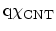eV Electron affinity of a CNT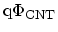eV Work function of a CNT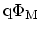eV Work function of the metal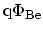eV Energy barrier height for electrons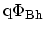eV Energy barrier height for holes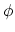V Electrostatic potential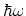eV Phonon energy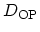eV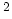Electron-optical-phonon coupling coefficient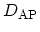eVElectron-acoustic-phonon coupling coefficient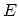eV Energy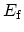eV FERMI energy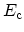eV Conduction band edge energy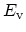eV Valence band edge energy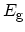eV Band gap energy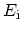eV Intrinsic energy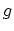m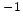eVDensity of states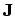A Current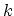mWave number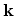mWave number vector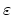AsVmDielectric permittivity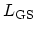m Gate-source spacer length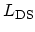m Drain-source space lengthkg Mass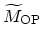eV/Å Reduced matrix elements of electron-optical-phonon interaction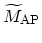eV Reduced matrix elements of electron-acoustic-phonon interaction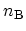1 Bose-EINSTEIN distribution function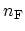1 FERMI-DIRAC distribution function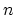mElectron concentration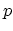mHole concentration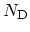mConcentration of donors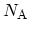mConcentration of acceptors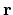m Space vector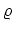mCarrier concentration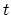s Time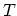K Temperature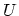eV Potential energy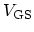V Gate-source voltage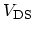V Drain-source voltage

M. Pourfath: Numerical Study of Quantum Transport in Carbon Nanotube-Based Transistors